Skip to content
Related Articles
HeapSort
• Difficulty Level : Medium
• Last Updated : 18 May, 2021

Heap sort is a comparison-based sorting technique based on Binary Heap data structure. It is similar to selection sort where we first find the minimum element and place the minimum element at the beginning. We repeat the same process for the remaining elements.

What is Binary Heap?
Let us first define a Complete Binary Tree. A complete binary tree is a binary tree in which every level, except possibly the last, is completely filled, and all nodes are as far left as possible (Source Wikipedia)
A Binary Heap is a Complete Binary Tree where items are stored in a special order such that the value in a parent node is greater(or smaller) than the values in its two children nodes. The former is called max heap and the latter is called min-heap. The heap can be represented by a binary tree or array.

Why array based representation for Binary Heap?
Since a Binary Heap is a Complete Binary Tree, it can be easily represented as an array and the array-based representation is space-efficient. If the parent node is stored at index I, the left child can be calculated by 2 * I + 1 and the right child by 2 * I + 2 (assuming the indexing starts at 0).

Heap Sort Algorithm for sorting in increasing order:
1. Build a max heap from the input data.
2. At this point, the largest item is stored at the root of the heap. Replace it with the last item of the heap followed by reducing the size of heap by 1. Finally, heapify the root of the tree.
3. Repeat step 2 while the size of the heap is greater than 1.

How to build the heap?
Heapify procedure can be applied to a node only if its children nodes are heapified. So the heapification must be performed in the bottom-up order.
Lets understand with the help of an example:

```Input data: 4, 10, 3, 5, 1
4(0)
/   \
10(1)   3(2)
/   \
5(3)    1(4)

The numbers in bracket represent the indices in the array
representation of data.

Applying heapify procedure to index 1:
4(0)
/   \
10(1)    3(2)
/   \
5(3)    1(4)

Applying heapify procedure to index 0:
10(0)
/  \
5(1)  3(2)
/   \
4(3)    1(4)
The heapify procedure calls itself recursively to build heap
in top down manner.```

## C++

 `// C++ program for implementation of Heap Sort``#include ` `using` `namespace` `std;` `// To heapify a subtree rooted with node i which is``// an index in arr[]. n is size of heap``void` `heapify(``int` `arr[], ``int` `n, ``int` `i)``{``    ``int` `largest = i; ``// Initialize largest as root``    ``int` `l = 2 * i + 1; ``// left = 2*i + 1``    ``int` `r = 2 * i + 2; ``// right = 2*i + 2` `    ``// If left child is larger than root``    ``if` `(l < n && arr[l] > arr[largest])``        ``largest = l;` `    ``// If right child is larger than largest so far``    ``if` `(r < n && arr[r] > arr[largest])``        ``largest = r;` `    ``// If largest is not root``    ``if` `(largest != i) {``        ``swap(arr[i], arr[largest]);` `        ``// Recursively heapify the affected sub-tree``        ``heapify(arr, n, largest);``    ``}``}` `// main function to do heap sort``void` `heapSort(``int` `arr[], ``int` `n)``{``    ``// Build heap (rearrange array)``    ``for` `(``int` `i = n / 2 - 1; i >= 0; i--)``        ``heapify(arr, n, i);` `    ``// One by one extract an element from heap``    ``for` `(``int` `i = n - 1; i > 0; i--) {``        ``// Move current root to end``        ``swap(arr, arr[i]);` `        ``// call max heapify on the reduced heap``        ``heapify(arr, i, 0);``    ``}``}` `/* A utility function to print array of size n */``void` `printArray(``int` `arr[], ``int` `n)``{``    ``for` `(``int` `i = 0; i < n; ++i)``        ``cout << arr[i] << ``" "``;``    ``cout << ``"\n"``;``}` `// Driver code``int` `main()``{``    ``int` `arr[] = { 12, 11, 13, 5, 6, 7 };``    ``int` `n = ``sizeof``(arr) / ``sizeof``(arr);` `    ``heapSort(arr, n);` `    ``cout << ``"Sorted array is \n"``;``    ``printArray(arr, n);``}`

## Java

 `// Java program for implementation of Heap Sort``public` `class` `HeapSort {``    ``public` `void` `sort(``int` `arr[])``    ``{``        ``int` `n = arr.length;` `        ``// Build heap (rearrange array)``        ``for` `(``int` `i = n / ``2` `- ``1``; i >= ``0``; i--)``            ``heapify(arr, n, i);` `        ``// One by one extract an element from heap``        ``for` `(``int` `i = n - ``1``; i > ``0``; i--) {``            ``// Move current root to end``            ``int` `temp = arr[``0``];``            ``arr[``0``] = arr[i];``            ``arr[i] = temp;` `            ``// call max heapify on the reduced heap``            ``heapify(arr, i, ``0``);``        ``}``    ``}` `    ``// To heapify a subtree rooted with node i which is``    ``// an index in arr[]. n is size of heap``    ``void` `heapify(``int` `arr[], ``int` `n, ``int` `i)``    ``{``        ``int` `largest = i; ``// Initialize largest as root``        ``int` `l = ``2` `* i + ``1``; ``// left = 2*i + 1``        ``int` `r = ``2` `* i + ``2``; ``// right = 2*i + 2` `        ``// If left child is larger than root``        ``if` `(l < n && arr[l] > arr[largest])``            ``largest = l;` `        ``// If right child is larger than largest so far``        ``if` `(r < n && arr[r] > arr[largest])``            ``largest = r;` `        ``// If largest is not root``        ``if` `(largest != i) {``            ``int` `swap = arr[i];``            ``arr[i] = arr[largest];``            ``arr[largest] = swap;` `            ``// Recursively heapify the affected sub-tree``            ``heapify(arr, n, largest);``        ``}``    ``}` `    ``/* A utility function to print array of size n */``    ``static` `void` `printArray(``int` `arr[])``    ``{``        ``int` `n = arr.length;``        ``for` `(``int` `i = ``0``; i < n; ++i)``            ``System.out.print(arr[i] + ``" "``);``        ``System.out.println();``    ``}` `    ``// Driver code``    ``public` `static` `void` `main(String args[])``    ``{``        ``int` `arr[] = { ``12``, ``11``, ``13``, ``5``, ``6``, ``7` `};``        ``int` `n = arr.length;` `        ``HeapSort ob = ``new` `HeapSort();``        ``ob.sort(arr);` `        ``System.out.println(``"Sorted array is"``);``        ``printArray(arr);``    ``}``}`

## Python

 `# Python program for implementation of heap Sort` `# To heapify subtree rooted at index i.``# n is size of heap`  `def` `heapify(arr, n, i):``    ``largest ``=` `i  ``# Initialize largest as root``    ``l ``=` `2` `*` `i ``+` `1`     `# left = 2*i + 1``    ``r ``=` `2` `*` `i ``+` `2`     `# right = 2*i + 2` `    ``# See if left child of root exists and is``    ``# greater than root``    ``if` `l < n ``and` `arr[largest] < arr[l]:``        ``largest ``=` `l` `    ``# See if right child of root exists and is``    ``# greater than root``    ``if` `r < n ``and` `arr[largest] < arr[r]:``        ``largest ``=` `r` `    ``# Change root, if needed``    ``if` `largest !``=` `i:``        ``arr[i], arr[largest] ``=` `arr[largest], arr[i]  ``# swap` `        ``# Heapify the root.``        ``heapify(arr, n, largest)` `# The main function to sort an array of given size`  `def` `heapSort(arr):``    ``n ``=` `len``(arr)` `    ``# Build a maxheap.``    ``for` `i ``in` `range``(n``/``/``2` `-` `1``, ``-``1``, ``-``1``):``        ``heapify(arr, n, i)` `    ``# One by one extract elements``    ``for` `i ``in` `range``(n``-``1``, ``0``, ``-``1``):``        ``arr[i], arr[``0``] ``=` `arr[``0``], arr[i]  ``# swap``        ``heapify(arr, i, ``0``)`  `# Driver code``arr ``=` `[``12``, ``11``, ``13``, ``5``, ``6``, ``7``]``heapSort(arr)``n ``=` `len``(arr)``print``(``"Sorted array is"``)``for` `i ``in` `range``(n):``    ``print``(``"%d"` `%` `arr[i]),``# This code is contributed by Mohit Kumra`

## C#

 `// C# program for implementation of Heap Sort``using` `System;` `public` `class` `HeapSort {``    ``public` `void` `sort(``int``[] arr)``    ``{``        ``int` `n = arr.Length;` `        ``// Build heap (rearrange array)``        ``for` `(``int` `i = n / 2 - 1; i >= 0; i--)``            ``heapify(arr, n, i);` `        ``// One by one extract an element from heap``        ``for` `(``int` `i = n - 1; i > 0; i--) {``            ``// Move current root to end``            ``int` `temp = arr;``            ``arr = arr[i];``            ``arr[i] = temp;` `            ``// call max heapify on the reduced heap``            ``heapify(arr, i, 0);``        ``}``    ``}` `    ``// To heapify a subtree rooted with node i which is``    ``// an index in arr[]. n is size of heap``    ``void` `heapify(``int``[] arr, ``int` `n, ``int` `i)``    ``{``        ``int` `largest = i; ``// Initialize largest as root``        ``int` `l = 2 * i + 1; ``// left = 2*i + 1``        ``int` `r = 2 * i + 2; ``// right = 2*i + 2` `        ``// If left child is larger than root``        ``if` `(l < n && arr[l] > arr[largest])``            ``largest = l;` `        ``// If right child is larger than largest so far``        ``if` `(r < n && arr[r] > arr[largest])``            ``largest = r;` `        ``// If largest is not root``        ``if` `(largest != i) {``            ``int` `swap = arr[i];``            ``arr[i] = arr[largest];``            ``arr[largest] = swap;` `            ``// Recursively heapify the affected sub-tree``            ``heapify(arr, n, largest);``        ``}``    ``}` `    ``/* A utility function to print array of size n */``    ``static` `void` `printArray(``int``[] arr)``    ``{``        ``int` `n = arr.Length;``        ``for` `(``int` `i = 0; i < n; ++i)``            ``Console.Write(arr[i] + ``" "``);``        ``Console.Read();``    ``}` `    ``// Driver code``    ``public` `static` `void` `Main()``    ``{``        ``int``[] arr = { 12, 11, 13, 5, 6, 7 };``        ``int` `n = arr.Length;` `        ``HeapSort ob = ``new` `HeapSort();``        ``ob.sort(arr);` `        ``Console.WriteLine(``"Sorted array is"``);``        ``printArray(arr);``    ``}``}` `// This code is contributed``// by Akanksha Rai(Abby_akku)`

## PHP

 ` ``\$arr``[``\$largest``])``        ``\$largest` `= ``\$l``;` `    ``// If right child is larger than largest so far``    ``if` `(``\$r` `< ``\$n` `&& ``\$arr``[``\$r``] > ``\$arr``[``\$largest``])``        ``\$largest` `= ``\$r``;` `    ``// If largest is not root``    ``if` `(``\$largest` `!= ``\$i``)``    ``{``        ``\$swap` `= ``\$arr``[``\$i``];``        ``\$arr``[``\$i``] = ``\$arr``[``\$largest``];``        ``\$arr``[``\$largest``] = ``\$swap``;` `        ``// Recursively heapify the affected sub-tree``        ``heapify(``\$arr``, ``\$n``, ``\$largest``);``    ``}``}` `// main function to do heap sort``function` `heapSort(&``\$arr``, ``\$n``)``{``    ``// Build heap (rearrange array)``    ``for` `(``\$i` `= ``\$n` `/ 2 - 1; ``\$i` `>= 0; ``\$i``--)``        ``heapify(``\$arr``, ``\$n``, ``\$i``);` `    ``// One by one extract an element from heap``    ``for` `(``\$i` `= ``\$n``-1; ``\$i` `> 0; ``\$i``--)``    ``{``        ``// Move current root to end``        ``\$temp` `= ``\$arr``;``            ``\$arr`` = ``\$arr``[``\$i``];``            ``\$arr``[``\$i``] = ``\$temp``;` `        ``// call max heapify on the reduced heap``        ``heapify(``\$arr``, ``\$i``, 0);``    ``}``}` `/* A utility function to print array of size n */``function` `printArray(&``\$arr``, ``\$n``)``{``    ``for` `(``\$i` `= 0; ``\$i` `< ``\$n``; ++``\$i``)``        ``echo` `(``\$arr``[``\$i``].``" "``) ;``        ` `}` `// Driver program``    ``\$arr` `= ``array``(12, 11, 13, 5, 6, 7);``    ``\$n` `= sizeof(``\$arr``)/sizeof(``\$arr``);` `    ``heapSort(``\$arr``, ``\$n``);` `    ``echo` `'Sorted array is '` `. ``"\n"``;``    ` `    ``printArray(``\$arr` `, ``\$n``);` `// This code is contributed by Shivi_Aggarwal``?>`

## Javascript

 ``
Output
```Sorted array is
5 6 7 11 12 13 ```

Here is previous C code for reference.

Notes:
Heap sort is an in-place algorithm.
Its typical implementation is not stable, but can be made stable (See this)

Time Complexity: Time complexity of heapify is O(Logn). Time complexity of createAndBuildHeap() is O(n) and the overall time complexity of Heap Sort is O(nLogn).

Applications of HeapSort
1. Sort a nearly sorted (or K sorted) array
2. k largest(or smallest) elements in an array
Heap sort algorithm has limited uses because Quicksort and Mergesort are better in practice. Nevertheless, the Heap data structure itself is enormously used. See Applications of Heap Data Structure
https://youtu.be/MtQL_ll5KhQ
Snapshots: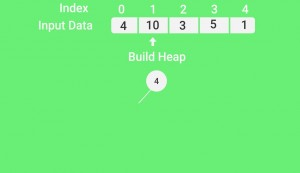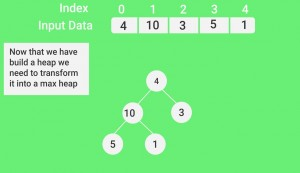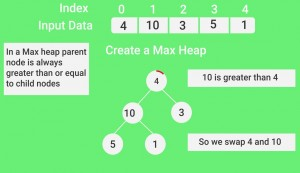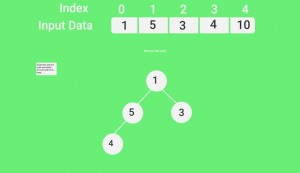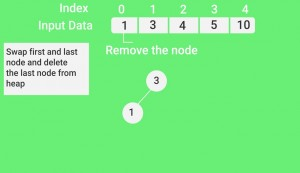## Quiz on Heap Sort

Other Sorting Algorithms on GeeksforGeeks/GeeksQuiz:
QuickSort, Selection Sort, Bubble Sort, Insertion Sort, Merge Sort, Heap Sort, QuickSort, Radix Sort, Counting Sort, Bucket Sort, ShellSort, Comb Sort, Pigeonhole Sort

Coding practice for sorting.

Please write comments if you find anything incorrect, or you want to share more information about the topic discussed above.

Attention reader! Don’t stop learning now. Get hold of all the important DSA concepts with the DSA Self Paced Course at a student-friendly price and become industry ready.  To complete your preparation from learning a language to DS Algo and many more,  please refer Complete Interview Preparation Course.

In case you wish to attend live classes with industry experts, please refer DSA Live Classes

My Personal Notes arrow_drop_up